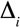### J. Wilson White and Robert R. Warner. 2007. Safety in numbers and the spatial scaling of density-dependent mortality in a coral reef fish. Ecology 88:3044–3054.

Appendix H. A summary of the model selection for the site-scale mortality analysis.

Comparison of multiple regression models for large scale instantaneous mortality rate based on monthly surveys of recruit and subadult density in 2004. Covariates are settler density (D), predator abundance (P) and habitat rugosity (R). AIC difference () is the difference between the AICc of model i and the lowest AICc observed. Akaike weight (wi) is calculated as the model likelihood, exp(-/ 2), normalized by the sum of all model likelihoods; values close to 1 indicate greater confidence in the selection of a model.

 Modelwi D 0 0.843 P 18.5 < 0.001 R 18.4 < 0.001 D, P 4.7 0.081 D, R 5.2 0.063 D, P, D × P 11.4 0.003 D, R, D × R 11.7 0.002 R, P 22.1 < 0.001 R, P, R × P 24.9 < 0.001 D, P, R 9.7 0.007 D, P, R, D × P 17.1 < 0.001 D, P, R, D × R 14.4 < 0.001 D, P, R, R × P 17.5 < 0.001 D, P, R, D × P, R × P 26.9 < 0.001 D, P, R, R × P, D × R 26.5 < 0.001 D, P, R, D × P, D × R 27.9 < 0.001 D, P, R, D × P, R × P, D × R 47.5 < 0.001

[Back to E088-189]